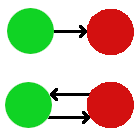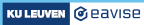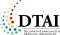2. Defining the situations

# 2. Defining the situations

A situation consists of a set of atomic objects and a number of relations/functions on these objects. The objects can be divided into different kinds, which are called types. A vocabulary defines which types, relations, and functions there are. For instance, the following two pictures show situations in the domain of graphs.

To describe such situations, we can use the following vocabulary

This vocabulary consists of a single type Node and a function edge that maps each pair `(x,y)` of nodes to true or false. Intuitively, `edge(x,y) = True` if and only if there is an edge from `x` to `y`. Each structure for this vocabulary consists of a specific set of Node objects, and of such an edge function, that represents a set of edges. In other words, there is a one-to-one correspondence between graphs and structures for this vocabulary.

The two example graphs above correspond to the following structures:

### Exercise

Here is a picture of another graph:

Which structure for the vocabulary GraphVoc corresponds to this graph? Try to write it yourself. Click on the Run button to check if you got it right.

Some terminology:

• Predicates/relations: a function with Bool as its range can be seen as representing a set of tuples, just like a table in a relational database. We also call such a function a predicate or a relation.
• Built-in types: The vocabulary GraphVoc makes use of a type Bool, which it does not explicitly declare. Bool is an example of a built-in type, that is always available for use in any vocabulary. Other built-in types are: Int, Real and Date, representing integers, real numbers and dates.

We now consider coloured graphs. In a coloured graph, there are also objects of type Colour and each node object is mapped to a colour object.

### Exercise

Here is a picture of a coloured graph, which uses the colours Red, Green and Blue.

Which structure for the vocabulary ColouredGraphVoc corresponds to this graph?

Choosing an appropriate vocabulary is a bit of an art. Often, there are different alternatives and it is not clear which is the best one. To illustrate, here is an alternative way of representing graphs:

Here, the edges are also explicit objects, i.e., each edge has its own identity. The graph shown at the start of this chapter can be represented by the following structure for this alternative vocabulary:

Is this alternative vocabulary better or worse than our original one? Here are some considerations:

• It is more complex, in the sense that it introduces more types and more functions.
• It allows us to represent also graphs in which there is more than one edge between the same pair of nodes. In other words, this vocabulary allows us to distinguish between these two graphs:The choice between the vocabularies may boil down to the question of whether a particular application needs this distinction or not.

• It may be easier to add additional properties to the edges in the alternative vocabulary. For instance, in route planning applications each edge typically has a weight. This can be elegantly added to the alternative vocabulary as follows:

In general, the following guidelines may be of use when choosing a vocabulary:

• Simplicity is good. If we can get away with fewer types and functions, then why not.
• A one-to-one mapping between the situations we want to consider and the structures for our vocabulary is convenient, if we can achieve it.

### Aside: partial functions

Can we also add weights to graphs that are represented by our original vocabulary GraphVoc? One idea is to replace the function

`edge: Node * Node -> Bool`

with:

`edge: Node * Node -> Int`

However, the problem with this is that a structure must now assign an integer value to each pair `(x,y)` of nodes. For a pair `(x,y)` that actually corresponds to an edge in the graph, this is not a problem. But what with pairs `(x,y)` for which there isn't an edge from `x` to `y` in the graph? In this case, there is no weight that we can associate with `(x,y)`.

In mathematical terms, the problem here is that we have declared a total function Edge, i.e., one which assigns a value to each tuple in its domain, while in reality the weight function of a graph is a partial function: it is only defined for those pairs `(x,y)` such that there is an edge from `x` to `y`; for all other pairs, it is undefined. Future versions of FO(·) might include support for such partial functions.

###### Made with love (and Hugo) by Joost Vennekens and Simon Vandevelde. :-)